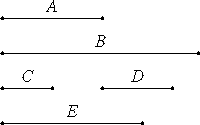# Proposition 11

Between two square numbers there is one mean proportional number, and the square has to the square the duplicate ratio of that which the side has to the side.

Let A and B be square numbers, and let C be the side of A, and D of B.

I say that between A and B there is one mean proportional number, and A has to B the ratio duplicate of that which C has to D.

Multiply C by D to make E.

Now, since A is a square and C is its side, therefore C multiplied by itself makes A. For the same reason also, D multiplied by itself makes B.VII.17

Since, then, C multiplied by the numbers C and D makes A and E respectively, therefore C is to D as A is to E.

VII.18

For the same reason also C is to D as E is to B. Therefore A is to E as E is to B. Therefore between A and B there is one mean proportional number.

I say next that A also has to B the ratio duplicate of that which C has to D.

V.Def.9

Since A, E, and B are three numbers in proportion, therefore A has to B the ratio duplicate of that which A has to E.

But A is to E as C is to D, therefore A has to B the ratio duplicate of that which the side C has to D.

Therefore, between two square numbers there is one mean proportional number, and the square has to the square the duplicate ratio of that which the side has to the side.

Q.E.D.

## Guide

Between c2 and d2 is the mean proportional cd, and the ratio c2 : d2 is the duplicate ratio of c : d. The argument for the latter statement is that c2 : d2 is compounded of the two ratios c2 : cd and cd : d2, but both of those are the same ratio as c : d.

#### Use of this proposition

This proposition is used in propositions VIII.14, VIII.15, and X.9.